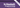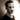Recursion!This is the weapon of a functional programmer. Not as clumsy or random as a for-loop; an elegant weapon for a more civilized age.

Taking a step back from a pretty dense chapter on more functional patterns, this time we're looking specifically at one thing: recursion. Chapter 8 of the book covers this in pretty good detail, giving a fairly wide range of examples, but I'll be trying to distill it down to its essence in this post. Let's start at the very beginning, why don't we?

## What is recursion?

A recursive function is---in its simplest terms---a function which calls itself. As the book describes it: "recursion is defining a function in terms of itself via self-referential expressions." This means that until some condition is met, the function will keep calling itself. If this condition is never met, the function never terminates. Recursion is an important concept in Haskell, because it gives us a way to express indefinite computations.

### Base cases

To reiterate: given that it terminates, a recursive function is a function that will keep calling itself until some condition to stop recursing is met. This condition is often known as the base case. For instance, let's look at writing a factorial function, which is a function that multiplies all positive integers (${1..n}$) up to and including the number we're calculating for. In maths, it's commonly written using an exclamation point, such as $4!$.

So we're all on the same page about what the function should do, $4!$ is evaluated as $4 \cdot 3 \cdot 2 \cdot 1$, which is equal to $24$.

To make this a recursive function, we know that for any number $n$ greater than one, it is the result of the factorial of $n-1$ multiplied by $n$. In other words, for $n$ greater than one, $n! = n \cdot (n-1)!$. If $n$ is $1$, then $n! = 1$. In our case, the function is not defined for integers less than one.

Based on the little analysis above, we now know that the value of $n!$ is recursively dependent on the value of $(n-1)!$ and so on. We have also identified our base case: $1$. So how would we go about writing this out? How about something like this:

factorial :: Integral a => a -> a
factorial 1 = 1
factorial n = n * factorial (n - 1)

Note that this function does not terminate if applied to a value less than $1$. This is an issue that we'll come back to shortly.

### Recursion as indefinite function composition

One thing that the book mentions that I found quite interesting, is that you can think of recursion as indefinite function composition. When composing functions, all we do is pipe the output of one function into another function. Recursion is the same thing, except it's always the same function. We can use this to create functions that will get applied an arbitrary number of times. Indeed, taking it a step further, we can write a function that takes a function, an argument, and the number of times to apply it, and then applies the function the set number of times:

applyNTimes :: (Integral a) => a -> (b -> b) -> b -> b
applyNTimes 0 _ b = b
applyNTimes n f b = f . applyNTimes (n - 1) f \$ b

Using this, we can create an increment function:

increment :: (Integral a, Num b) => a -> b -> b
increment times = applyNTimes times (+1)
-- increment 5 2 = 7

A contrived example, but it's a pretty cool effect!

## Bottom

I mentioned when talking about the factorial function that it doesn't terminate for every possible value we can apply it to. A function that never terminates is one of the ways we can play with bottoms in Haskell (and no, it's not a kinky as it sounds!).

In Haskell, bottom (symbolically: ⊥) is used to refer to computations that do not result in a value. The two main varieties of bottom are computations that fail with an error (partial functions) and computations that fail to terminate (such as with infinite recursion and the factorial example above). When you can, stay away from bottom.

## Definitons

This chapter only comes with a single definition: recursion. The funny thing would be to tell you to start from the top, but let's be boring and do it the proper way:

Recursion
Recursion is a means of computing results that may require an indefinite amount of work to obtain through the use of repeated function application. A recursive function will usually have at least one case that calls itself and a base case that stops the recursion.Thomas Heartman is a developer, writer, speaker, and one of those odd people who enjoy lifting heavy things and putting them back down again. Preferably with others. Doing his best to gain and share as much knowledge as possible.